# Preface

This tutorial was made solely for the purpose of education and it was designed for students taking Applied Math 0340. It is primarily for students who have some experience using Mathematica. If you have never used Mathematica before and would like to learn more of the basics for this computer algebra system, it is strongly recommended looking at the APMA 0330 tutorial. As a friendly reminder, don't forget to clear variables in use and/or the kernel. The Mathematica commands in this tutorial are all written in bold black font, while Mathematica output is in regular fonts.

Finally, you can copy and paste all commands into your Mathematica notebook, change the parameters, and run them because the tutorial is under the terms of the GNU General Public License (GPL). You, as the user, are free to use the scripts for your needs to learn the Mathematica program, and have the right to distribute this tutorial and refer to this tutorial as long as this tutorial is accredited appropriately. The tutorial accompanies the textbook Applied Differential Equations. The Primary Course by Vladimir Dobrushkin, CRC Press, 2015; http://www.crcpress.com/product/isbn/9781439851043

Introduction to Linear Algebra

# Numerical Solutions

p2 = Show[
ParametricPlot[
Table[{x[t], y[t]} /. sol[[j]], {j, Length[ic]}], {t, 0, T},
PlotRange -> {{0, 4}, {0, 3}}],
Arrow[{ic[[j]], ic[[j]] + .01 f[ic[[j, 1]], ic[[j, 2]] ] }]}],
{j, Length[ic]}],
AxesLabel -> {"x", "y"},
BaseStyle -> {FontFamily -> "Times", FontSize -> 14},
PlotLabel -> "Phase portrait"
]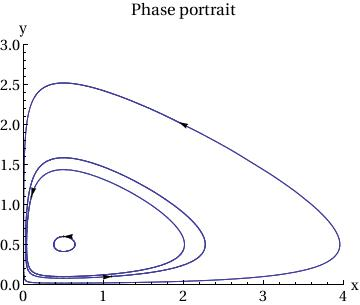Plot[{x[t] /. sol[], y[t] /. sol[]}, {t, 0, T},
PlotStyle -> {{Thickness[0.01], RGBColor[1, 0, 0]}, {Thickness[0.01],
RGBColor[0, 1, 0]}}, AxesOrigin -> {0, 0},
AxesLabel -> {"t", "x(t),y(t)"},
BaseStyle -> {FontFamily -> "Times", FontSize -> 14},
PlotLabel -> "Time courses: Red - x(t), Green - y(t)"]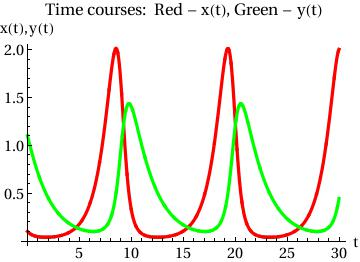Example. Consider the system of autonomous equations

$\dot{x} = x^2 -3\,xy, \qquad \dot{y} = 2xy-y^2 .$
First, we plot the direction field using StreamPLot command:
StreamPlot[{x^2 - 3*x*y, 2*x*y - y^2}, {x, -3, 3}, {y, -3, 3}]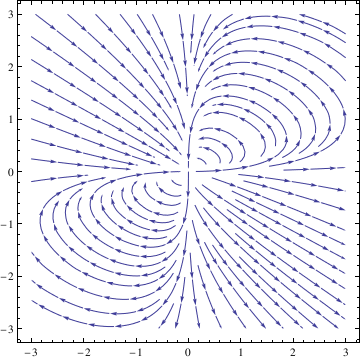Then we can use NDSolve function to solve the given system numerically subject to some initial conditions:
deq1 = x'[t] == x[t]^2 - 3*x[t]*y[t];
deq2 = y'[t] == 2*x[t]*y[t] - y[t]^2;
soln = NDSolve[{deq1, deq2, x == 1, y == 1}, {x[t], y[t]}, {t, -10, 10}];
Next, we plot the corresponding solutions curve with the command
x = soln[[1, 1, 2]]; y = soln[[1, 2, 2]]; ParametricPlot[{x, y}, {t, -10, 10}, PlotStyle -> Thick]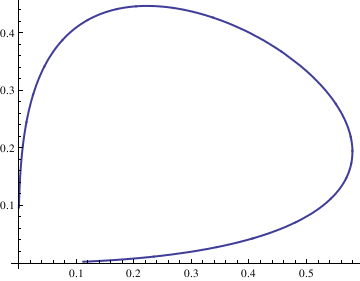We can plot as many such solutions curves as we want, and then display them simultaneously. For example, the following command creates a list of solution curves corresponding to the initial conditions $$x(0) = n/10, \quad y(0) =n/10$$ for $$n=-4,-3,\ldots , 3,4.$$
curve = Table[n, {n, -4, 4}]; curvep = Table[n, {n, 0, 4}];
Do[Clear[x, y];
soln = NDSolve[{deq1, deq2, x == n/10, y == n/10}, {x[t], y[t]}, {t, -10, 10}];
x = soln[[1, 1, 2]];
y = soln[[1, 2, 2]];
curve[[n]] = ParametricPlot[{x, y}, {t, -10, 10}, PlotStyle -> Thick], {n, -4, 4}];
Show[curve[[-4]], curve[[-3]], curve[[-2]], curve[[-1]], curve[], curve[], curve[], curve[], AspectRatio -> 1]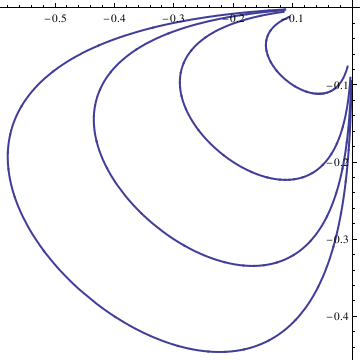To display solutions in the first quadrant, we type:
Do[Clear[x, y];
soln = NDSolve[{deq1, deq2, x == n/10, y == n/10}, {x[t], y[t]}, {t, -10, 10}];
x = soln[[1, 1, 2]];
y = soln[[1, 2, 2]];
curvep[[n]] = ParametricPlot[{x, y}, {t, -10, 10}, PlotStyle -> Thick], {n, 0, 4}];
Show[curvep[], curvep[], curvep[], curvep[], curvep[], AspectRatio -> 1]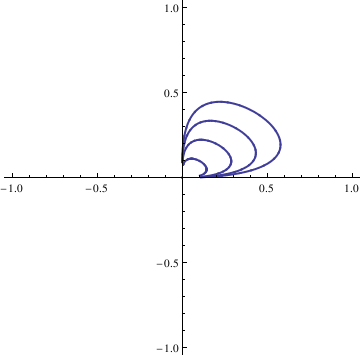F[x_, y_] := -y^3;
G[x_, y_] := x^3;
sol = NDSolve[{x'[t] == F[x[t], y[t]], y'[t] == G[x[t], y[t]],
x == 1, y == 0}, {x, y}, {t, 0, 3*Pi},
WorkingPrecision -> 20]
ParametricPlot[Evaluate[{x[t], y[t]}] /. sol, {t, 0, 3*Pi}]

X[t_] := Evaluate[x[t] /. sol]
Y[t_] := Evaluate[y[t] /. sol]
fns[t_] := {X[t], Y[t]};
len := Length[fns[t]];
Plot[Evaluate[fns[t]], {t, 0, 3*Pi}]